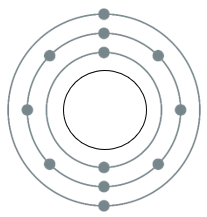### Question 3. An element has its electron configuration as 2,8,2. Now answer the following questions. a. What is the atomic number of this element? b. What is the group of this element? c. To which period does this element belong?

Question 3.

An element has its electron configuration as 2,8,2. Now answer the following questions.

a. What is the atomic number of this element?

b. What is the group of this element?

c. To which period does this element belong?

d. With which of the following elements would this element resemble? (Atomic numbers are given in the brackets)

N (7), Be (4), Ar (18), Cl (17)

Answer:

a. Atomic number of the given element is 12.

Explanation:

Atomic number of the element is equal to the total number of protons present in it. In the given element:

Total number of protons = 2 + 8 + 2

= 12

b. The group of this element is 2.

Explanation:

The number of electrons present in the outermost shell of the element is 2. This means its valency is 2.

Thus, it belongs to the group 2.

c. This element belongs to period 3.

Explanation:

The element belongs to period 3 because it has total 3 shells (2,8,2). The number of shells tell the period number of the element.d. Among the given elements, Be would be the element to which the given element resembles

Explanation:

The electronic configuration of Be is 2,2. The number of electrons present in the outermost shell is 2. Thus, Be belongs to the group 2 (alkaline earth metals). The given element also belongs to group 2.

Thus, Be resembles the given element.